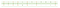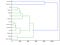# Using the Needleman-Wunsch algorithm to draw evolutionary trees

This is a story I have written a while back while I was still doing some Bioinformatics related work. Nowadays this is a field that is constantly growing and evolving so keeping up with it if you’re not part of this world is quite a time consuming task. I am hoping this piece will inspire people that are interested into the field to dive into it!

The Needleman–Wunsch algorithm performs a global alignment on two genetic sequences. It is commonly used in bioinformatics to align protein or nucleotide sequences. It is also an example of dynamic programming, and was the first application of dynamic programming to biological sequence comparison. It is sometimes referred to as the Optimal matching algorithm.

Before we start drawing evolutionary trees, we need to align the sequences. Before we start aligning them, lets discuss what alignment is. Sequence alignment is probably the most important task in bioinformatics; it is routinely applied to both amino acid and DNA sequences. The ultimate purpose of sequence alignment is to measure sequence similarity, or how closely sequences resemble each other. It is only by first aligning sequences, that we can say whether an amino acid or nucleotide is the same or different between sequences, and hence how similar the sequences are over their entire length. Lets take a simple alignment example (fictional) just to show an example on how alignment looks like.Global alignment of two fictional sequences — VIVALASVEGAS and VIVADAVIS.

Here we have two fictional sequences, VIVALASVEGAS and VIVADAVIS. The picture represents a global alignment.

A global alignment of two sequences,

and

is an asignment of gap symbols “-” into those sequences, or at their ends. The two resulting strings are placed one above the other so that every character or gap symbol in either string is opposite a unique character or a unique gap symbol in the other string. It can be represented as a

matrix for some value of

the first row containing the first sequence and (interspersed) gap symbols, the second row containing the second sequence and (interspersed) gap symbols. Note that

the total length of the alignment will be either equal to the length of the longest sequence or longer if gaps have been inserted, but cannot be longer than

since insertion of gap symbols at the same position in both rows is considered meaningless.

But our goal is not to find a global alignment, but an optimal global alignment. We need to find the best possible arrangement. For that we use scoring functions. Every position in the alignment,

specifies a pair of opposing symbols from an expanded alphabet of nucleotides or amino acids that include the gap symbol (“-“). Let

and

be the symbols that are appearing at the i-th position of the alignment. A scoring function is denoted by

Once we define the scoring function for pairs of symbols from that alphabet, the score of the alignment between sequences, M, can be defined as an additive function of the symbol scores. So if we have a function

characterizing the cost of aligning two symbols

and

then we can define a function to compute the cost of an entire alignment as follows:

where we sum over all positions of the alignment. We won’t go into details, but if you are interested I highly recommend the book Introduction to computational genomics A case studies approach. The choice of a scoring function is crucial in determining the score of the alignment, and this function should also be reflective of biological constraints.

A convenient way of representing scoring functions is a substitution matrix. It shows the cost of replacing one letter (of either, the nucleotide or amino acid alphabet) with another letter or a gap. In our example, we’ll be using the BLOSUM50 substitution matrix:

Save the contents above to the file BLOSUM50.txt. Lets write the code that reads the BLOSUM50 matrix:

Ok, some code is written, lets continue with the theory now. We can now define what an optimal global alignment is.

The optimal (global) alignment of two strings,

and

is defined as the alignment

that maximizes the total alignment score over all possible alignments. The optimal alignment is often referred to as

and

is its alignment score.

The naive approach in finding the optimal global alignment is calculating scores for all possible alignments and ranking them. The number of different alignments (with gaps) of two sequences of length

is

Fortunately, two nice people came up with an algorithm to solve this problem, and the answer is dynamic programming and the use of the Needleman-Wunsch algorithm. The algorithm can be written as the following recursion:

In words, the score of the alignment between

and

is equal to the maximum of the score of the alignment of the three prefixes plus the score for the consequent edit operation. We have all we need, now let’s write the code.

As you can see, the function receives two sequences, the blosum matrix and a penalty (number) as the input. Ok, now lets get some sequences from the GENBANK. So that you don’t have to download them, we’ll implement this in code:

As you can see we are reading the COX3 gene and we are putting them in the organisms table. Now that we’ve done this, we need to calculate a scoring matrix between organisms.

Basically, we are computing all the permutations between all the organisms and on each pair we run the Needleman-Wunsch algorithm. Then we store the score alignments in the scoring matrix. Now that that’s done, we need to calculate the scoring distance matrix:

In code that’s:

Now we just need to put things together:

We plot the dendrogram with the data we have calculated and our end result is the following figure:The result of our calculations — the dendrogram we were looking for.

And for those who didn’t want to read everything, here is the whole Python script:

## REFERENCES

1. N. Christianini, M. W. Hahn, Introduction to computational genomics A case studies approach, United states of America: Cambridge University Press, 2006, pages 43–46.
2. The Needleman-Wunsch algorithm. Online: http://en.wikipedia.org/wiki/Needleman-Wunsch algorithm
3. BLOSUM50 substitution matrix. Online: http://www.ncbi.nlm.nih.gov/IEB/ToolBox/C_DOC/lxr/source/data/BLOSUM50
4. COX-3. Online: http://en.wikipedia.org/wiki/COX-3

Javascript Engineer @PovioLabs, Professor @ Erudio Izobraževalni Center, Mentor @Thinkful and Instructor @SmartNinjaOrg

## More from Boštjan Cigan

Javascript Engineer @PovioLabs, Professor @ Erudio Izobraževalni Center, Mentor @Thinkful and Instructor @SmartNinjaOrg